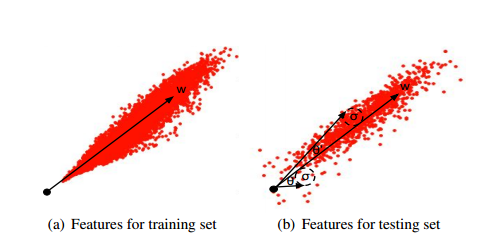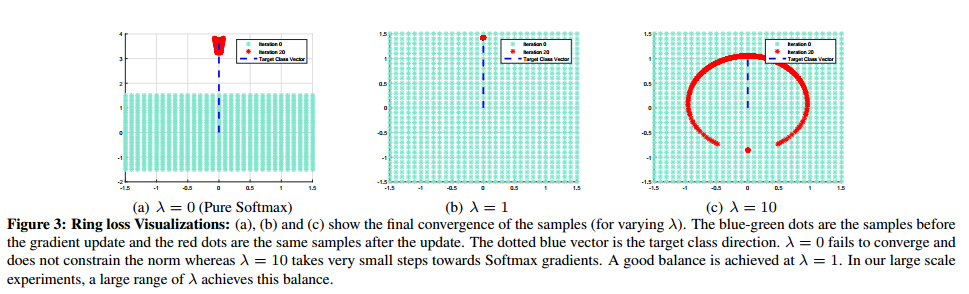## （原）Ring loss Convex Feature Normalization for Face Recognition

http://www.cnblogs.com/darkknightzh/p/8858998.html

Ring loss: Convex Feature Normalization for Face Recognition

https://arxiv.org/abs/1803.00130v1

Ring loss将特征限制到缩放后的单位圆上，同时能保持凸性，来得到更稳健的特征。$\min {{L}_{s}}(F(x))\text{ s}\text{.t}\text{. }{{\left\| F(x) \right\|}_{2}}=R$

R为归一化后特征的模。由于模的等式限制，导致实际上该损失函数是非凸的（这句话不太确定怎么翻译，反正是非凸）。

${{L}_{R}}=\frac{\lambda }{2m}\sum\limits_{i=1}^{m}{{{({{\left\| F({{x}_{i}}) \right\|}_{2}}-R)}^{2}}}$

$\frac{\partial {{L}_{R}}}{\partial R}=-\frac{\lambda }{m}\sum\limits_{i=1}^{m}{({{\left\| F({{x}_{i}}) \right\|}_{2}}-R)}$

$\frac{\partial {{L}_{R}}}{\partial F({{x}_{i}})}=\frac{\lambda }{m}(1-\frac{R}{{{\left\| F({{x}_{i}}) \right\|}_{2}}})F({{x}_{i}})$posted on 2018-04-16 22:34  darkknightzh  阅读(1537)  评论(0编辑  收藏  举报